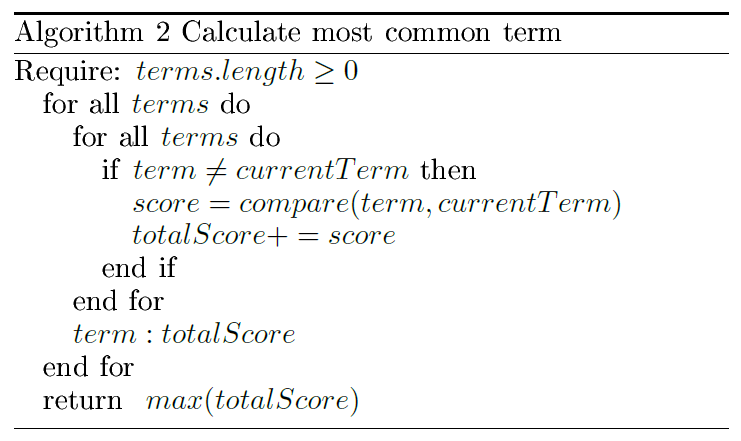top of page
Search
• Alibek Jakupov

# LDA automatic labeling

Updated: Nov 19, 2021As topic modeling has increasingly attracted interest from researchers there exists plenty of algorithms that produce a distribution over words for each latent topic (a linguistic one) and a distribution over latent topics for each document. The list consists of explicit Dirichlet Allocation  that incorporates a preexisting distribution based off Wikipedia; Concept-topic model (CTM)  where a multinomial distribution is placed over known concepts with associated word sets; Non-negative Matrix Factorization (https://medium.com/ml2vec/topic-modeling-is-an-unsupervised-learning-approach-to-clustering-documents-to-discover-topics-fdfbf30e27df) that, unlike the others, does not rely on probabilistic graphical modeling and factors high-dimensional vectors into a low-dimensionally representation. However, most of them are often based off Latent Dirichlet Allocation (LDA)  which is a state-of-the-art method for generating topics, so all of our experiments are based on LDA.

In LDA each document in the corpus (collection of documents) is represented as a multinomial distribution over topics. Each topic is represented as the multinomial distribution over words. Based on the likelihood it is possible to claim that only a small number of words are important. In this case the model simultaneously learns the topics by iteratively sampling topic assignment to every word in every document (in other words calculation of distribution over distributions), using the Gibbs sampling update.

As it has been mentioned above every topic is a multinomial distribution over terms. Consequently, a standard way of interpreting a topic is extracting top terms with the highest marginal probability (a probability that the terms belongs to a given topic). However, for tasks where the topics distributions are provided to humans as a first-order output, it may be difficult to interpret the rich statistical information encoded in the topics. In this work we apply several techniques and evaluate them on the real-word dataset.

The main approach consists in generating hypernyms for top terms. In linguistics a hypernym is a word or phrase whose semantic field is more general than that of another word in the same domain. For example, 'bird' is a hypernym for pigeon, crow and eagle. This approach is inspired by the natural attitude when the human tries to find the most generic term for the series of semantically related words. We first take each term in the topic and generate its hypernym using the Wordnet. Next, we calculate the number of common hypernyms and iteratively continue the process till the manually defined threshold is achieved.In addition, we find the most common term from the top terms using DBPedia. DBPedia is a Knowledge Graph whose knowledge is extracted from Wikipedia. Thus analyzing this graph we can calculate the semantic similarity between each two terms. In this work we applied Wu and Palmer similarity concept based on the depth of the Least Common Subsumer and Wordnet Taxonomies.We then combine both approaches and also add LOD concept thus adding common sense knowledge to the framework.

 J. A. Hansen, E. K. Ringger, and K. D. Seppi. Probabilistic explicit topic modeling using wikipedia. In I. Gurevych, C. Biemann, and T. Zesch, editors, Language Processing and Knowledge in theWeb, pages 69-82, Berlin, Heidelberg, 2013. Springer Berlin Heidelberg.

 P. S. Chaitanya Chemudugunta and M. Steyvers. Text modeling using unsupervised topic models and concept hierarchies. CoRR, abs/0808.0973, 2008.

 M. I. J. David M. Blei, Andrew Y. Ng. Latent Dirichlet Allocation. Journal of Machine Learning Research, 3, 2003.